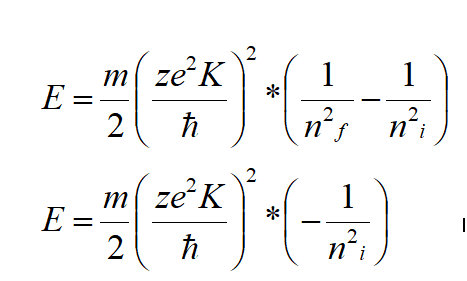# Why does this equation not give me the ionization energy of lithium?

• Chemistry
patric44
Homework Statement:
i want to find the first ionization energy for lithium using Bohr formula but something seems wrong !
Relevant Equations:
Bohr energy equation
i am trying to calculate the first ionization energy for lithium with this equation but it seems to work only with hydrogen ?
i put nf = infinity and ni =2 ,and z = 3 for lithium and it gives about 30 ev not 5.39172 ev which is the value for lithium :i will appreciate any help in this

Mentor
There are some limitations to the Bohr model, do you know them?

patric44
There are some limitations to the Bohr model, do you know them?
i know that it failed to describe the experimental results for multi electron atoms,
so what equation is being used for finding the ionization energy ?
because every where i look online it seems that people uses that equation ?!